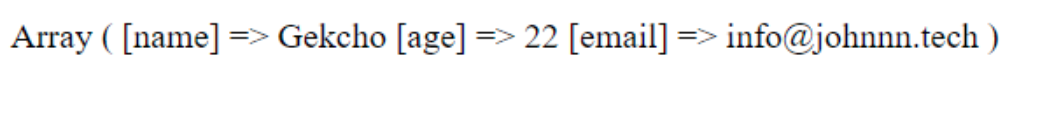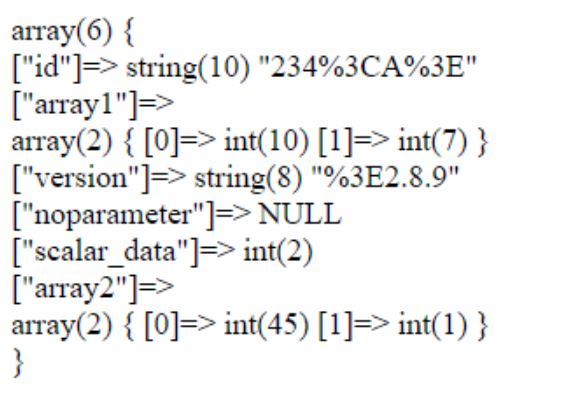# PHP | filter_input_array() Function

The filter_input_array() function is an inbuilt function in PHP which is used to get external variables (e.g. from form input) and filters them if it is specified. This function is similar to filter_input() function but the only difference is filter_input() filters a single value but in filter_input_array() filters the whole array according to options provided. It is useful for retrieving/filtering many values instead of calling filter_input() many times. This is new in PHP only works in PHP 5.2 or latter version of PHP.

Syntax:

`mixed filter_input_array( \$type_of_data, \$definition, \$add_empty_parameter )`

Parameters: This function accepts three parameters as mentioned above and described below:

• type_of_data: It is required parameter. It holds the input type of the data to check. The available options are:
• INPUT_GET
• INPUT_POST
• INPUT_SERVER
• INPUT_ENV
• definition: It is optional parameter. It specifies an array of filter arguments or parameters. A valid array key as a variable name and a valid value as a filter name or ID, or an array specifying the filter, flags, and options. This parameter can also be a single filter name/ID just like filter_input() then all values in the input array are filtered by the specified filter.
• add_empty_parameter: It is optional parameter. It is a Boolean parameter. It adds missing keys as NULL to the return value when it sets to True. Its default value is True.

Return Value: It returns an array containing the values of the variables on success and on failure, it returns False. If the input array designated by type is not populated, the function returns NULL if the FILTER_NULL_ON_FAILURE flag is not given, otherwise, it returns False. For other failures, False is returned.

Below programs illustrate the filter_input_array() function in PHP:

program 1:

 ` ``array``( ` `        ``"filter"` `=> FILTER_CALLBACK, ` `        ``"flags"` `=> FILTER_FORCE_ARRAY, ` `        ``"options"` `=> ``"ucwords"` `    ``), ` `    ``"age"` `=> ``array``( ` `        ``"filter"` `=> FILTER_VALIDATE_INT, ` `        ``"options"` `=> ``array``( ` `            ``"min_range"` `=> 1, ` `            ``"max_range"` `=> 120 ` `        ``) ` `    ``), ` `    ``"email"``=> FILTER_VALIDATE_EMAIL, ` `); ` ` `  `print_r(filter_input_array(INPUT_GET, ``\$filters``)); ` ` `  `?> `

Output:Note: This example may not give the expected result on online IDE as it doesn’t support the passing of parameters in GET or POST method. So, try to run it in some PHP hosting server or localhost and pass the values of the parameter either by GET or POST method.

Program 2:
Data came from POST method:

```\$_POST = array(
'product_id' => '234<A>',
'component'  => array('10'),
'version'    => '<2.8.9',
'array2'  => array('45', '1'),
'scalar_data' => '2',
);
```

 ` FILTER_SANITIZE_ENCODED, ` `    ``'array1'` `=> ``array``( ` `        ``'filter'` `=> FILTER_VALIDATE_INT, ` `        ``'flags'` `=> FILTER_REQUIRE_ARRAY,  ` `        ``'options'` `=> ``array``( ` `            ``'min_range'` `=> 1, ` `            ``'max_range'` `=> 10 ` `        ``) ` `    ``), ` `    ``'version'` `=> FILTER_SANITIZE_ENCODED, ` `    ``'noparameter'` `=> FILTER_VALIDATE_INT, ` `    ``'scalar_data'` `=> ``array``( ` `        ``'filter'` `=> FILTER_VALIDATE_INT, ` `        ``'flags'`  `=> FILTER_REQUIRE_SCALAR, ` `    ``), ` `    ``'array2'` `=> ``array``( ` `        ``'filter'` `=> FILTER_VALIDATE_INT, ` `        ``'flags'`  `=> FILTER_REQUIRE_ARRAY, ` `    ``) ` `); ` `   `  `\$allinputs` `= filter_input_array(INPUT_GET, ``\$args``); ` `   `  `var_dump(``\$allinputs``); ` `echo` `"\n"``; ` ` `  `?> `

Output:Note: This example may not give the expected result on online IDE as it doesn’t support the passing of parameters in GET or POST method. So, try to run it in some PHP hosting server or localhost and pass the values of the parameter either by GET or POST method.

My Personal Notes arrow_drop_upCheck out this Author's contributed articles.

If you like GeeksforGeeks and would like to contribute, you can also write an article using contribute.geeksforgeeks.org or mail your article to contribute@geeksforgeeks.org. See your article appearing on the GeeksforGeeks main page and help other Geeks.

Please Improve this article if you find anything incorrect by clicking on the "Improve Article" button below.

Article Tags :
Practice Tags :

Be the First to upvote.

Please write to us at contribute@geeksforgeeks.org to report any issue with the above content.